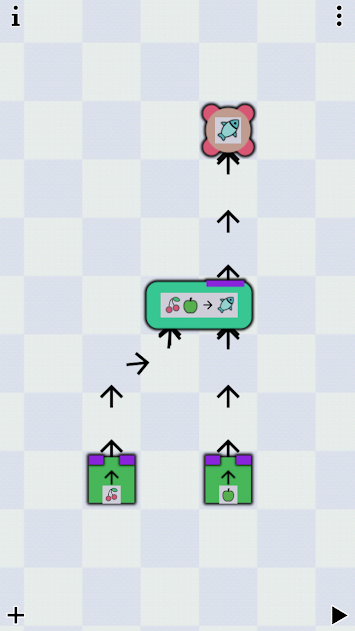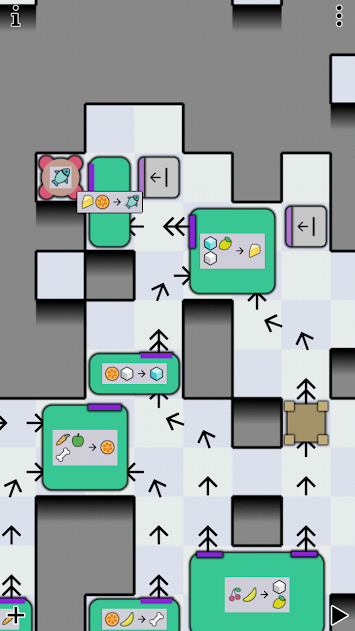Published: Fri 07 September 2018

# Finding Trade and Exchange Possibilities with Prolog

A friend recently had an interesting problem for me: There's a game for mobile phones where you have to exchange or transmute wares into each other, in order to create some strange supply chain.Example of an earlier level.A very complex later example.

The game is bleentoro, image copyright by them.

In this example (an earlier level) the goal is to create fish. We can transmute or exchange (I will only write about exchange from now on) an apple and a pair of cherries for a fish. Apple and cherry we have, or are created from thin air, in the context of the game. As you can see in the other example, this can get very complex.

The question is, given some input we have, in what order do we have to use the stations for exchange to create some output. How do we best model this problem?

## Modeling the Problem

From a previous article we already know that we can encode numbers in Prolog terms using Peano numbers. Using these we can match terms that represent at least or exactly some number. Exactly 3 would be s(s(s(z))) while at least 3 would be s(s(s(X))) with the variable X representing the rest of the term for some $$n$$ so that $$3 + x = n$$.

Let's start with a small example: we have an aunt that gives us apples. We have bob who is willing to exchange an apple for two berries. And we have a cousin who will bake a cake if we give them two apples and five berries.

Let's formalize our rules in some way:

a: / -> 1A
b: A -> 2B
c: 2A, 5B -> 1C


We model this as some kind of inventory(A, B, C) with some slots for Peano encoded numbers. Meaning that inventory(s(z), z, s(C)) means an inventory of exactly one apple, zero berries, and at least one cake. Lucky us.

Let's define our possible exchanges as Prolog facts with exchange(Kind, Pre, Post) meaning we use the exchange method Kind and we get from inventory state Pre to inventory state Post. If we lose an item, we have it in Pre but don't in Post. If we gain one, it's the other way around. Of course, the rest of our inventory should stay the same.

exchange(a, inventory(A, B, C), inventory(s(A), B, C)).
exchange(b, inventory(s(A),B,C), inventory(A, s(s(B)), C)).
exchange(c, inventory(s(s(A)), s(s(s(s(s(B))))), C), inventory(A, B, s(C))).


As we can see, we can encode basically any numeric condition that uses only "just exactly" and "at least", as well as addition and subtraction and "setting" fields to specific values. If we have to give at least three apples, but all of them, to get a cake, the rule would be exchange(c, inventory(s(s(s(_))), B, C), inventory(z, B, s(C)))..

## Programming the Solver

We want to create a predicate trades(Start, Goal, Path) where we give a Start inventory and a Goal inventory and want to get a Path of exchanges. The most obvious approach is the following:

trades(Goal, Goal, []).


So we're done when our Goal is reached. And if not, we have to try some exchange. We try it and we quickly learn that...

?- trades(inventory(z, z, z), inventory(_, _, s(z)), Path).
ERROR: Out of local stack


... it doesn't work.

The problem is, that calling the exchange predicate in the recursive call always tries the first rule first and since that basically always works, we get into an infinite loop. Let's reorder the rules and put the generating rule first.

?- trades(inventory(z, z, z), inventory(_, _, s(z)), Path).
ERROR: Out of local stack


It still doesn't work. Let's look at the trace.

[trace]  ?- trades(inventory(z, z, z), inventory(_, _, s(z)), Path).
Call: (8) trades(inventory(z, z, z), inventory(_700, _702, s(z)), _712) ? creep
Call: (9) exchange(_960, inventory(z, z, z), _984) ? creep
Exit: (9) exchange(a, inventory(z, z, z), inventory(s(z), z, z)) ? creep
Call: (9) trades(inventory(s(z), z, z), inventory(_700, _702, s(z)), _962) ? creep
Call: (10) exchange(_978, inventory(s(z), z, z), _1002) ? creep
Exit: (10) exchange(b, inventory(s(z), z, z), inventory(z, s(s(z)), z)) ? creep
Call: (10) trades(inventory(z, s(s(z)), z), inventory(_700, _702, s(z)), _980) ? creep
Call: (11) exchange(_1000, inventory(z, s(s(z)), z), _1024) ? creep
Exit: (11) exchange(a, inventory(z, s(s(z)), z), inventory(s(z), s(s(z)), z)) ? creep
Call: (11) trades(inventory(s(z), s(s(z)), z), inventory(_700, _702, s(z)), _1002) ? creep
Call: (12) exchange(_1018, inventory(s(z), s(s(z)), z), _1042) ? creep
Exit: (12) exchange(b, inventory(s(z), s(s(z)), z), inventory(z, s(s(s(s(z)))), z)) ? creep
Call: (12) trades(inventory(z, s(s(s(s(z)))), z), inventory(_700, _702, s(z)), _1020) ? creep
Call: (13) exchange(_1040, inventory(z, s(s(s(s(z)))), z), _1064) ? creep
Exit: (13) exchange(a, inventory(z, s(s(s(s(z)))), z), inventory(s(z), s(s(s(s(z)))), z)) ? creep
Call: (13) trades(inventory(s(z), s(s(s(s(z)))), z), inventory(_700, _702, s(z)), _1042) ? creep
Call: (14) exchange(_1058, inventory(s(z), s(s(s(s(z)))), z), _1082) ? creep
Exit: (14) exchange(b, inventory(s(z), s(s(s(s(z)))), z), inventory(z, s(s(s(s(s(s(z)))))), z)) ? creep
Call: (14) trades(inventory(z, s(s(s(s(s(s(z)))))), z), inventory(_700, _702, s(z)), _1060) ? creep
Call: (15) exchange(_1080, inventory(z, s(s(s(s(s(s(z)))))), z), _1104) ? creep
Exit: (15) exchange(a, inventory(z, s(s(s(s(s(s(z)))))), z), inventory(s(z), s(s(s(s(s(s(z)))))), z)) ? creep
Call: (15) trades(inventory(s(z), s(s(s(s(s(s(z)))))), z), inventory(_700, _702, s(z)), _1082) ? creep
Call: (16) exchange(_1098, inventory(s(z), s(s(s(s(s(s(z)))))), z), _1122) ? creep


We're still running into an infinite loop since we're just getting apples and trading them for berries.

There's a trick if our solution is a list. If the length(List, Length) predicate is called with both predicates free (length(L, _) for example), we get lists of increasing length as solutions. So if we can bind our solution to lists of increasing length, we won't get infinite loops of apple-berry-exchanges without ever trying short solutions.

So our query now looks like this:

?- length(Path,_), trades(inventory(z, z, z), inventory(_, _, s(z)), Path).
Path = [a, b, a, b, a, b, a, a, c] ;
Path = [a, b, a, b, a, a, b, a, c] ;
Path = [a, b, a, b, a, a, a, b, c] ;
Path = [a, b, a, a, b, b, a, a, c] ;
Path = [a, b, a, a, b, a, b, a, c] ;
Path = [a, b, a, a, b, a, a, b, c] ;
Path = [a, b, a, a, a, b, b, a, c] ;
Path = [a, b, a, a, a, b, a, b, c] ;
Path = [a, b, a, a, a, a, b, b, c] ...


As it turns out, there are a few ways to do it, but nothing shorter than 9 trades. We can even look, whether we have anything left over after we got our cake:

?- length(Path,_), trades(inventory(z, z, z), inventory(A, B, s(z)), Path).
Path = [a, b, a, b, a, b, a, a, c],
A = z,
B = s(z) .


Yeah. As it turns out, we have a berry to spare.

## Conclusion

This is how you do it. And of course, you could simulate a shop with that, as one inventory slot could be the number of coins. If the inventory gets too large, you can split it into item types so you don't have to copy every unchanged item from the pre-condition to the post-condition. Then you can copy a term that might represent any number of items.

But beware: this algorithm (with Prolog's evaluation scheme) has not so good complexity. For a solution of length $$n$$ and $$m$$ rules it has a run time of $$O(m^n)$$. We create every possible solution list permutation but break early, if no rules are applicable.

Depending on our instantiation of Start and Goal, this algorithm doesn't terminate (if the system isn't terminating, the algorithm isn't either). Our system doesn't terminate as we can always apply a again and again. We're creating increasingly longer partial solutions which might turn out to be no solution at all. If there is no solution, you won't know. The algorithm just tries longer and longer lists trying to find a solution.

If you have any other conditions on your solution (some exchanges might be limited or necessary) you can put most of them after the trades call. Some conditions, like membership, can be put before.

Let's say we have a terminating system by taking away the a rule. If our Start is fully instantiated, then our algorithm terminates. Let's try another query and find out, with how many apples we need to start to get a cake:

?- trades(inventory(A, z, z), inventory(_, _, s(z)), Path).
ERROR: Out of local stack


Through the unification rules, it is possible that a rule creates terms on the left side of the rule. In this case, the algorithm tries to give us increasing amounts of berries to match that with the starting condition (only rule b is applied in reverse, always leaving us with one cake, which doesn't match the starting condition). There we need the length predicate.

?- length(Path,_), trades(inventory(A, z, z), inventory(_, _, s(z)), Path).
Path = [b, b, b, c],
A = s(s(s(s(s(_2334))))) .


We need at least five apples for a cake. But we knew that.

To recap:

• We saw again how useful it is, to encode the natural numbers in a term structure.
• A solver is easily written but the algorithm isn't fast.
• The rules can be applied in both directions because of Prolog's evaluation scheme. This leaves queries that would intuitively work divergent.
• By using the length predicate we can simulate breadth-first evaluation, while the default evaluation scheme is depth-first.

I think, there's a different approach using linear programming. I might try that in the future.

Comments and Discussion is provided by Disqus. They are tracking site and user interaction. Please refer to their privacy policy for information about data usage and retention. If you still want to look at comments or comment yourself, enable disqus by clicking here.

Previous: Haskell Countdown Solver , Next: Java Primitive Types are bad for Learners